# Inductors Equations – What is Inductors and SymbolLike what we have learned about capacitors and resistors, now we will learn about what is inductors equations.

An inductor is a passive element designed to store energy in its magnetic field. Inductors find numerous applications in electronic and power systems. They are used in power supplies, transformers, radios, TVs, radars, and electric motors.

## What is Inductors and Symbol

Any conductor of electric current has inductive properties and may be regarded as an inductor. But in order to enhance the inductive effect, a practical inductor is usually formed into a cylindrical coil with many turns of conducting wire, as shown in Figure.(1).

An inductor consists of a coil of conducting wire.

If the current is allowed to pass through an inductor, it is found that the voltage across the inductor is directly proportional to the time rate of change of the current. Using the passive sign convention,

where L is the constant of proportionality called the inductance of the inductor. The unit of inductance is the henry (H), named in honor of the American inventor Joseph Henry (1797–1878). It is clear from Equation.(1) that 1 henry equals 1 volt-second per ampere.

Inductance is the property whereby an inductor exhibits opposition to the change of current flowing through it, measured in henrys (H).

The inductance of an inductor depends on its physical dimension and construction. Formulas for calculating the inductance of inductors of different shapes are derived from electromagnetic theory and can be found in standard electrical engineering handbooks. For example, for the inductor, (solenoid) shown in Figure.(1),

where N is the number of turns, ℓ is the length, A is the cross-sectional area, and μ is the permeability of the core. We can see from Equation.(2) that inductance can be increased by increasing the number of turns of coil, using a material with higher permeability as the core, increasing the cross-sectional area, or reducing the length of the coil.

Like capacitors, commercially available inductors come in different values and types. Typical practical inductors have inductance values ranging from a few microhenrys ( μH), as in communication systems, to tens of henrys (H) as in power systems.

Inductors may be fixed or variable. The core may be made of iron, steel, plastic, or air. The terms coil and choke are also used for inductors. Common inductors are shown in Figure.(2).

The circuit symbols for inductors are shown in Figure.(3), following the passive sign convention.

Equation.(1) is the voltage-current relationship for an inductor. Figure.(4) shows this relationship graphically for an inductor whose inductance is independent of the current. Such an inductor is known as a linear inductor.

For a nonlinear inductor, the plot of Equation.(1) will not be a straight line because its inductance varies with current. We will assume linear inductors in this post unless stated otherwise.

The current-voltage relationship is obtained from Equation.(1) asIntegrating gives

or

where i(t0) is the total current for −∞ < t < t0 and i(−∞) = 0. The idea of making i(−∞) = 0 is practical and reasonable because there must be time in the past when there was no current in the inductor.

The inductor is designed to store energy in its magnetic field. The energy stored can be obtained from Equation.(1). The power delivered to the inductor is

The energy stored is

Since i( −∞) = 0,

We should note the following important properties of an inductor.

1. Note from Equation.(1) that the voltage across an inductor is zero when the current is constant. Thus,

An inductor acts like a short circuit to dc.

2. An important property of the inductor is its opposition to the change in the current flowing through it.

The current through an inductor cannot change instantaneously.

According to Equation.(1), a discontinuous change in the current through an inductor requires an infinite voltage, which is not physically possible. Thus, an inductor opposes an abrupt change in the current through it.

For example, the current through an inductor may take the form shown in Figure.(5a), whereas the inductor current cannot take the form shown in Figure.(5b) in real-life situations due to the discontinuities. However, the voltage across an inductor can change abruptly.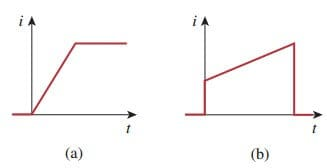Figure 5. Current through an inductor: (a) allowed, (b) not allowable; an abrupt change is not possible.

3. Like the ideal capacitor, the ideal inductor does not dissipate energy. The energy stored in it can be retrieved at a later time. The inductor takes power from the circuit when storing energy and delivers power to the circuit when returning previously stored energy.

4. A practical, nonideal inductor has a significant resistive component, as shown in Figure.(6). This is due to the fact that the inductor is made of a conducting material such as copper, which has some resistance.

This resistance is called the winding resistance Rw, and it appears in series with the inductance of the inductor. The presence of Rw makes it both an energy storage device and an energy dissipation device. Since Rw is usually very small, it is ignored in most cases.

The nonideal inductor also has a winding capacitance Cw due to the capacitive coupling between the conducting coils. Cw is very small and can be ignored in most cases, except at high frequencies. We will assume ideal inductors in this post.

## Inductors Equations Examples

1. The current through a 0.1-H inductor is
i(t) = 10te −5t A.
Find the voltage across the inductor and the energy stored in it.

Solution:
Since v = L didt and L = 0.1 H,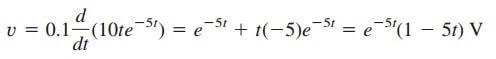The energy stored is2. Find the current through a 5-H inductor if the voltage across it isAlso, find the energy stored at t = 5 s. Assume i(v) > 0.

Solution:

Since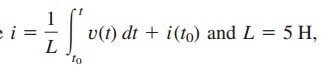Then,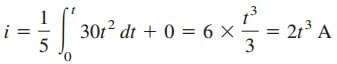The power p = vi = 60t5, and the energy stored is thenAlternatively, we can obtain the energy stored using Equation.(7), by writingas obtained before.

3. Consider the circuit in Figure.(7a). Under dc conditions, find:
(a) i, vC, and iL,
(b) the energy stored in the capacitor and inductor.

Solution:
(a) Under dc conditions, we replace the capacitor with an open circuit and the inductor with a short circuit, as in Figure.(7b). It is evident from Figure.(7b) thatThe voltage vC is the same as the voltage across the 5-Ω resistor. Hence,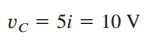(b) The energy in the capacitor is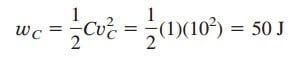and that in the inductor is